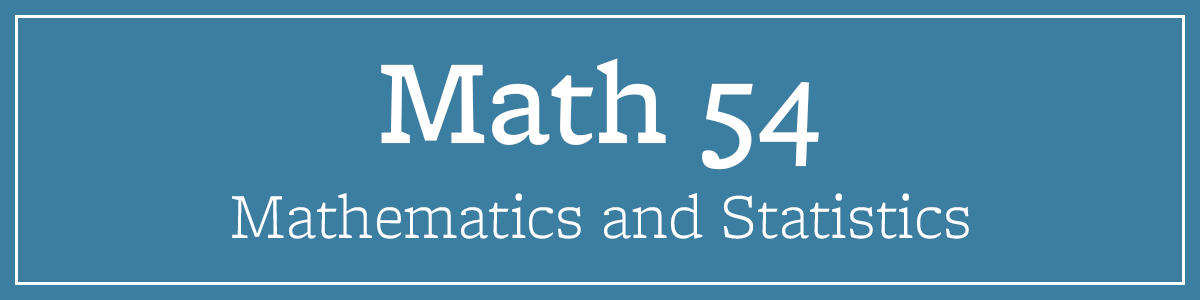# Math 54## Course Name

Linear Algebra and Differential Equations

## Course Description

Basic linear algebra; matrix arithmetic and determinants. Vector spaces; inner product spaces. Eigenvalues and eigenvectors; orthogonality, symmetric matrices. Linear second-order differential equations; first-order systems with constant coefficients. Fourier series, application to partial differential equations.